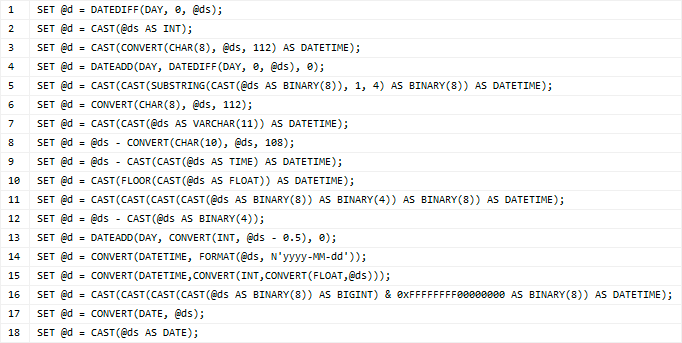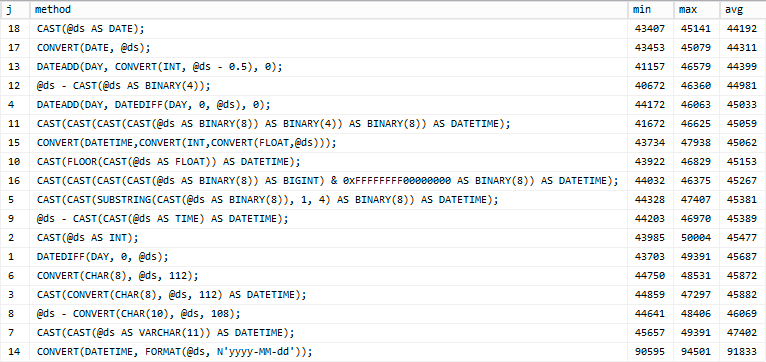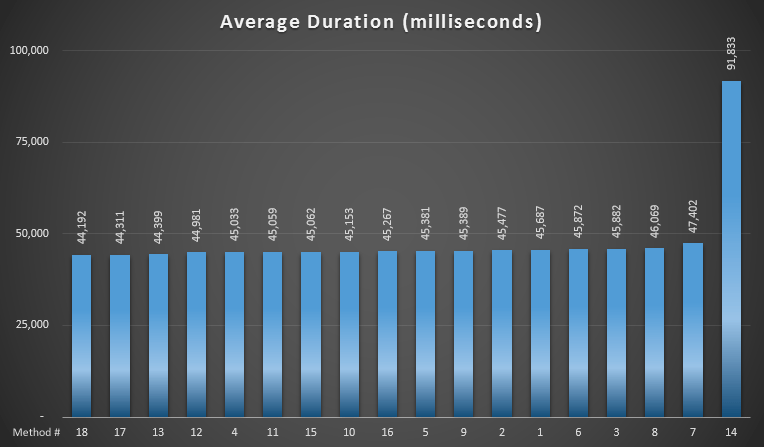# Trimming time from datetime – a follow-up

SentryOne eBooks

In these books, you will find useful, hand-picked articles that will help give insight into some of your most vexing performance problems. These articles were written by several of the SQL Server industry’s leading experts, including Paul White, Paul Randal, Jonathan Kehayias, Erin Stellato, Glenn Berry, Aaron Bertrand, and Joe Sack.

Featured AuthorPaul Randal, CEO of SQLskills, writes about knee-jerk performance tuning, DBCC, and SQL Server internals.

Paul’s Posts

Following up on my previous post about trimming time from datetime, I was spurred to demonstrate more clearly the performance characteristics of various methods without involving data access. In the original post, I quickly compared seven different methods of converting a datetime value to a date independently, showed that the differences were negligible, then moved straight into analyzing the use of those methods in actual queries that return data.

In this post I wanted to show several different ways to trim time from datetime (18 different ways in fact!), without introducing any actual data, to see if we could proclaim a "fastest" way to perform this task.

## The Methods

Here are the 18 methods I would be testing, some taken from the blog post Madhivanan pointed out after my previous post:

`DECLARE @d DATETIME, @ds DATETIME = SYSDATETIME();`## The Test

I created a loop where I would run each conversion 1,000,000 times, and then repeat the process for all 18 conversion methods 10 times. This would provide metrics for 10,000,000 conversions for each method, eliminating any significant statistical skew.

```CREATE TABLE #s(j INT, ms INT);
GO
SET NOCOUNT ON;
GO
DECLARE @j INT = 1, @x INT, @i INT = 1000000;
DECLARE @t DATETIME2, @d DATETIME, @ds DATETIME = SYSDATETIME();

WHILE @j <= 18
BEGIN
SELECT @x = 1, @t = SYSDATETIME();

WHILE @x <= @i
BEGIN
IF @j = 1
SET @d = DATEDIFF(DAY, 0, @ds);
IF @j = 2
SET @d = CAST(@ds AS INT);
IF @j = 3
SET @d = CAST(CONVERT(CHAR(8), @ds, 112) AS DATETIME);
IF @j = 4
SET @d = DATEADD(DAY, DATEDIFF(DAY, 0, @ds), 0);
IF @j = 5
SET @d = CAST(CAST(SUBSTRING(CAST(@ds AS BINARY(8)), 1, 4)
AS BINARY(8)) AS DATETIME);
IF @j = 6
SET @d = CONVERT(CHAR(8), @ds, 112);
IF @J = 7
SET @d = CAST(CAST(@ds AS VARCHAR(11)) AS DATETIME);
IF @J = 8
SET @d = @ds - CONVERT(CHAR(10), @ds, 108);
IF @J = 9
SET @d = @ds - CAST(CAST(@ds AS TIME) AS DATETIME);
IF @J = 10
SET @d = CAST(FLOOR(CAST(@ds AS FLOAT)) AS DATETIME);
IF @J = 11
SET @d = CAST(CAST(CAST(CAST(@ds AS BINARY(8)) AS BINARY(4))
AS BINARY(8)) AS DATETIME);
IF @J = 12
SET @d = @ds - CAST(@ds AS BINARY(4));
IF @J = 13
SET @d = DATEADD(DAY, CONVERT(INT, @ds - 0.5), 0);
IF @J = 14
SET @d = CONVERT(DATETIME, FORMAT(@ds, N'yyyy-MM-dd'));
IF @J = 15
SET @d = CONVERT(DATETIME,CONVERT(INT,CONVERT(FLOAT,@ds)));
IF @J = 16
SET @d = CAST(CAST(CAST(CAST(@ds AS BINARY(8)) AS BIGINT) &
0XFFFFFFFF00000000 AS BINARY(8)) AS DATETIME);
IF @J = 17
SET @d = CONVERT(DATE, @ds);
IF @j = 18
SET @d = CAST(@ds AS DATE);

SET @x += 1;
END

INSERT #s SELECT @j, DATEDIFF(MILLISECOND, @t, SYSDATETIME());

SET @j += 1;
END
GO 10

SELECT
j, method = CASE ... END,
MIN(ms), MAX(ms), AVG(ms)
FROM #s
GROUP BY j ORDER BY j;```

## The Results

I ran this on a Windows 8 VM, with 8 GB RAM and 4 vCPUs, running SQL Server 2012 (11.0.2376). Here are tabular results, sorted by average duration, fastest first:And here is a graphical representation of the average duration:If we removed the outlier (which uses SQL Server 2012's new `FORMAT` function, an obvious dog for this purpose), we'd have a real hard time picking a true loser here. Of the remaining 17 methods, to perform this conversion a million times, the slowest method is only three seconds slower than the fastest method, on average. The evidence still supports my earlier assertion that using `CAST / CONVERT` natively is about as efficient as you can get, but the improvement over the other approaches is only marginal, and it wasn't even the winner in every single run.